Courses
Courses for Kids
Free study material
Offline Centres
More

# What is Flat in Maths?Last updated date: 06th Dec 2023
Total views: 160.2k
Views today: 3.60k## Flat Meaning for Kids

In Mathematics, flat means smooth, even, or plane. For example, plane shapes, two-dimensional shapes, etc.

Let us look at a few geometrical shapes that are flat to understand what is flat in Maths in a better way.

## What Are Flat Shapes in Maths?

There are two types of shapes in Maths namely two-dimensional shapes and three-dimensional shapes. The flat shapes are two-dimensional shapes having only two dimensions i.e. length and width but no thickness. Flat shapes can be drawn on any plane paper, or flat surface.

Square, rectangle, circle, diamond, and triangle are some of the flat shapes in Maths.

Let us learn about these flat shapes in brief.

### Square

A square is a two-dimensional flat shape that has four equal sides and four vertices. The flat area enclosed inside the square is known as the square region.

Example:

The wall or the table that has all four sides equal and square area enclosed inside this is an example of a square.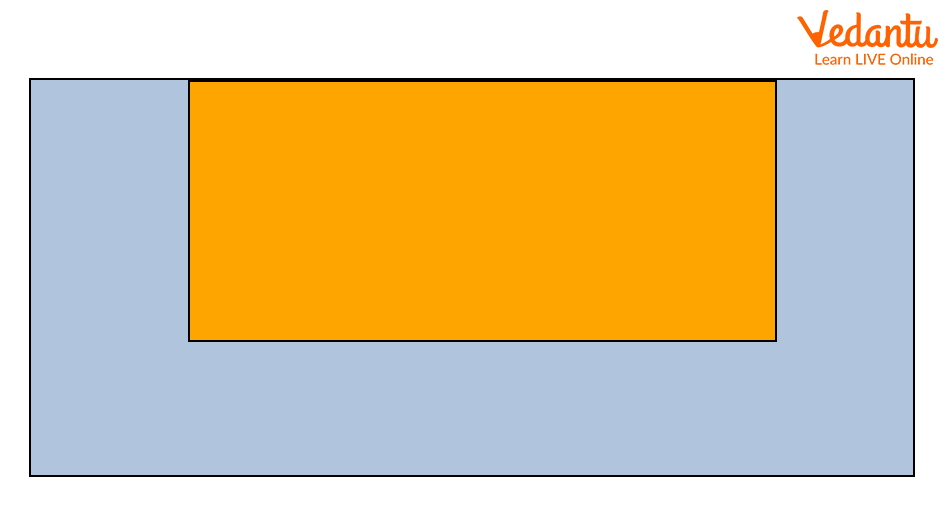Wall in square shape

### Rectangle

A rectangle is a two-dimensional flat shape whose opposite sides are equal and parallel to each other. The flat area enclosed inside the rectangle is known as the rectangular region.

Example:

The blackboard, rupee note, dollar notes, etc are some examples of rectangle shape.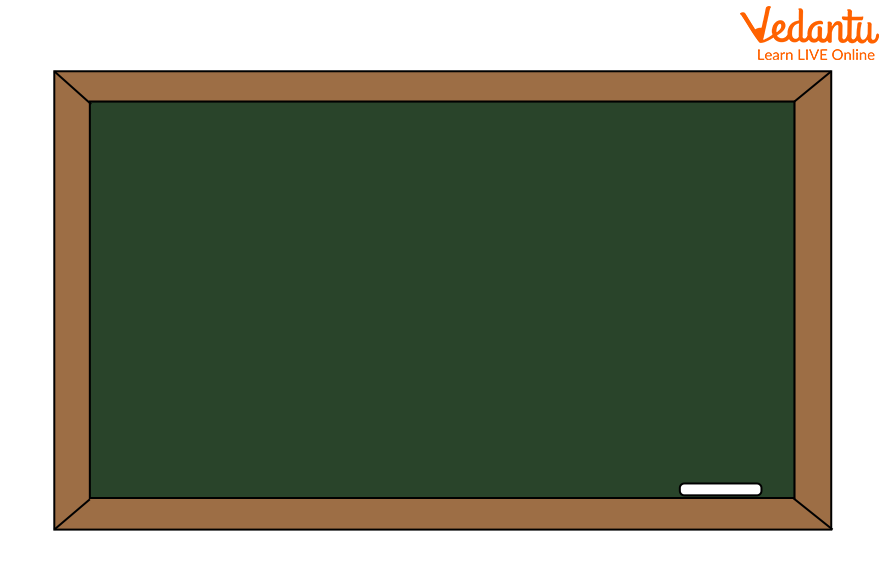Blackboard in rectangle shape

Triangle

A triangle is a three sided polygonal flat shape that has three sides and three vertices. The flat area enclosed inside the triangle is known as the triangular region.

Example:

Pyramids are one of the best examples of triangular shapes.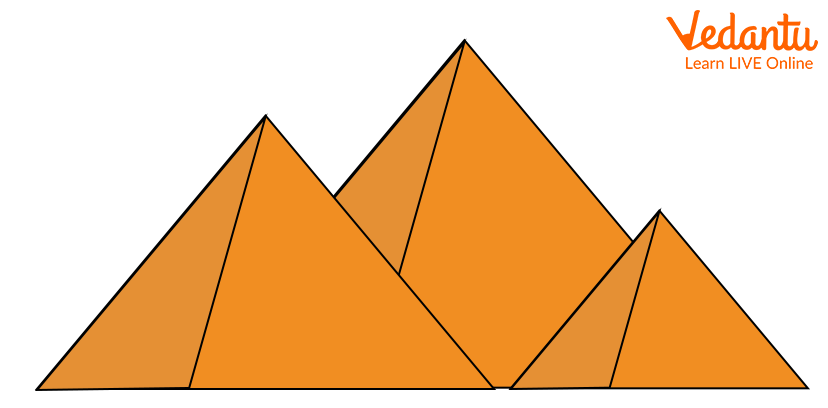Pyramids in triangular shape

### Circle

The circle is a two-dimensional flat shape that has only a curved boundary. The circle has no sides and corners. The area enclosed in the circle is known as a circular region.

Example:

The disk, pizza, wall clock, etc. are some examples of circular shape.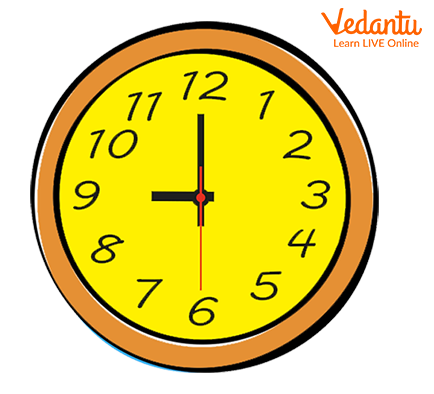Wall clock in circular shape

### Pentagon

The pentagon is a two-dimensional flat shape. It is a five-sided polygon. The flat area enclosed inside the pentagon is known as the pentagonal region.

Example:

The U.S. Department of Defence Headquarters is a perfect example of pentagonal shape.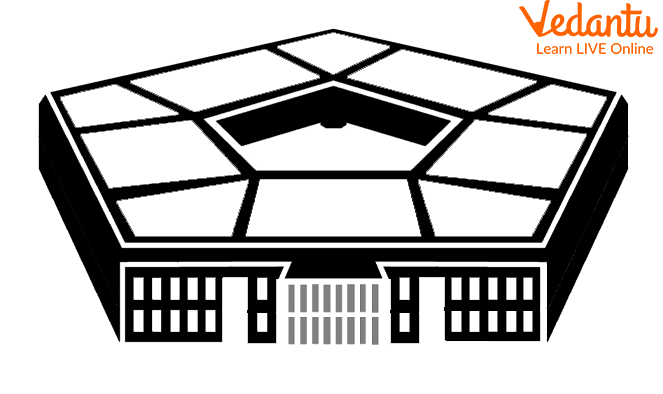A building in Pentagon shape

### Octagon

The octagon is a two-dimensional flat shape. It is an eight-sided polygon. The flat area enclosed inside the octagon is known as the octagonal region.

Example

The stop sign board on the roadside is a perfect example of octagon shape.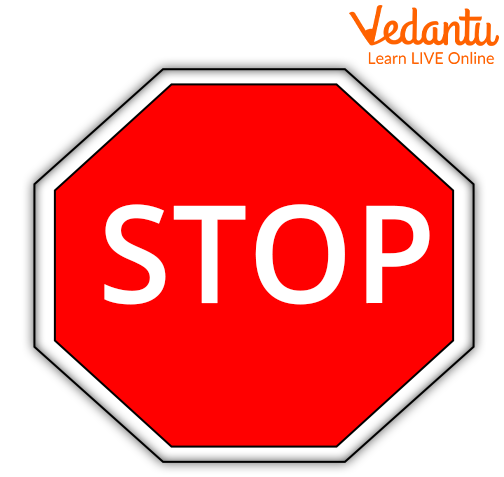Stop sign board in octagon shape

## Other Solid Shapes with Flat Faces

### Cube

A cube has 6 flat faces, 12 edges and 8 corners (vertices). Every face of a cube is square in shape. All sides of a cube are equal.

Example:

Rubix cube, sugar cubes, dice etc., are a few of the examples of the cube.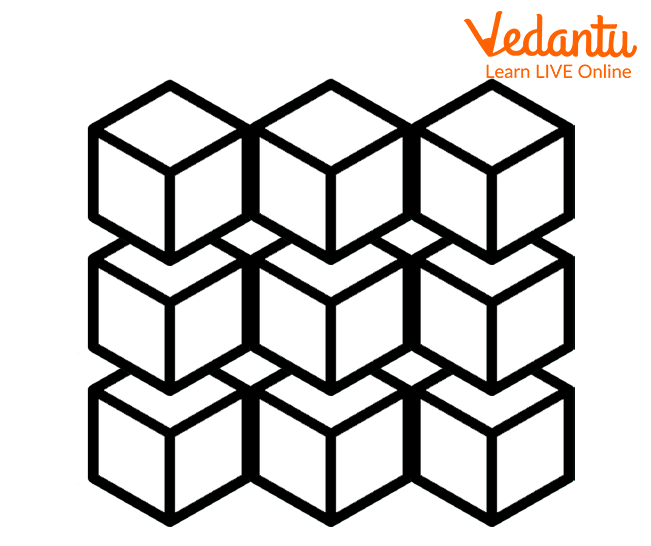Sugar Cubes

### Cuboid

A cuboid has 6 flat faces, 12 edges and 8 corners (vertices). Every face of a cuboid is rectangular in shape. Opposite sides of a cuboid are equal.

Example:

Shoeboxes, bricks, books etc., are a few examples of the cuboid.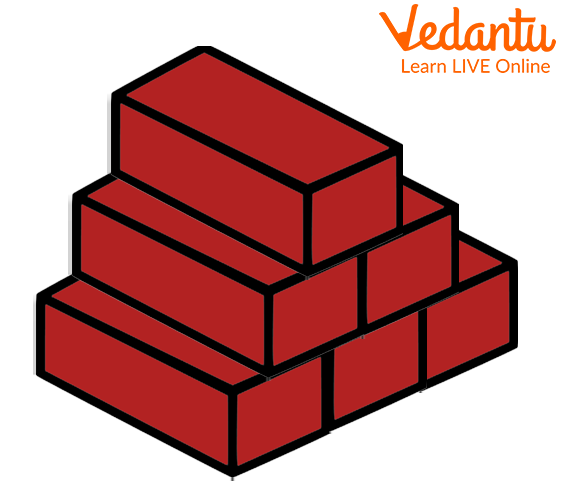A cuboid shape bricks

### Cone

A cone has one flat face and one curved face. It also has one edge and one corner (vertex).

Example

Party hats, traffic cones, ice cream cones etc, christmas trees, are a few of the examples of the cone shapes.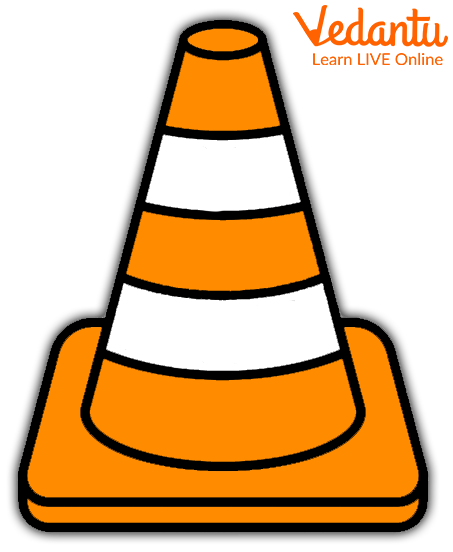A cone shape traffic cones

### Cylinder

A cylinder is a solid object with two similar flat ends that are circular or elliptical and one curved surface. Note: The cylinder has a flat base and a flat top. The base is the same as the top. The shape of a cylinder from base to top stays the same.

Example:

Water cans, candles, beakers, test tubes, etc., are a few examples of the cylinder.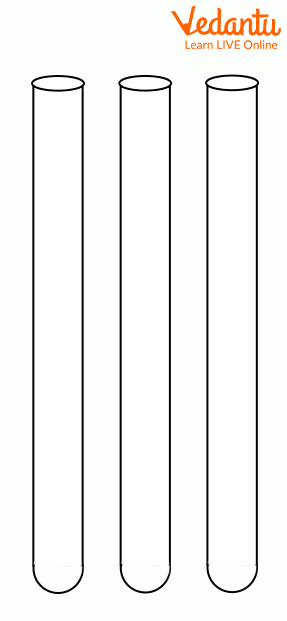Beaker in cylindrical shape

## Conclusion

In short, a smooth or even surface with no depth is termed as flat shape or flat surface. There are several two dimensional shapes in geometry that have a flat surface. Few of them include triangles, squares, rectangles, etc. Also, we have shapes such as cones, cylinders, etc. with flat faces. With this article, you will know how many flat faces solid shapes have.

## FAQs on What is Flat in Maths?

1. What are known as flat faces in Maths?

In Maths, a flat face is any of the individual flat surfaces of a solid object. For example, a cylinder has two flat faces, both are circular.

2. Is the plane shape a flat shape?

Yes, a plane is a flat or two-dimensional shape that has no thickness and is closed. Square, rectangle, triangle, circle, etc. are some example flat or plane shapes.